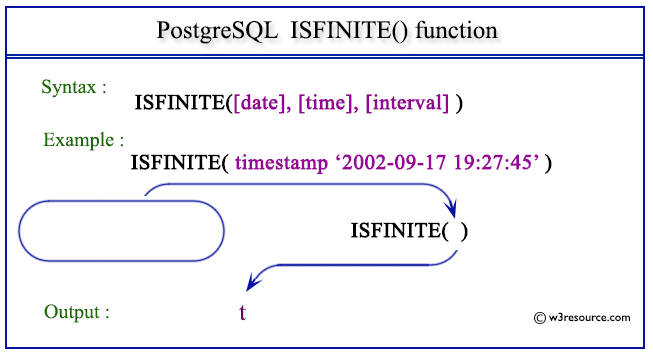# PostgreSQL ISFINITE() function

## ISFINITE() function

The isfinite() function is used to get test for finite date (not +/-infinity).

Syntax:

```isfinite(date)
or
isfinite(timestamp)
or
isfinite(interval)
```

Return Type: boolean.

PostgreSQL Version: 9.3

Pictorial Presentation of PostgreSQL ISFINITE() functionExample 1: PostgreSQL ISFINITE() function

Code:

``````SELECT isfinite(date '2002-09-17');
```
```

Sample Output:

```  isfinite
----------
t
(1 row)
```

Example 2: PostgreSQL ISFINITE() function

The isfinite() function is used to get the test for a finite date (not +/-infinity).

Code:

``````SELECT isfinite(timestamp '2002-09-17 19:27:45');
```
```

Sample Output:

``` isfinite
----------
t
(1 row)
```

Example 3: PostgreSQL ISFINITE() function

This function is used to test for a finite interval.

Code:

``````SELECT isfinite(interval '7 hours');
```
```

Sample Output:

```   isfinite
----------
t
(1 row)
```

Previous: EXTRACT function
Next: JUSTIFY_DAYS function

﻿